Next: Application of the Huber Up: Robust inversion using the Previous: Introduction

# Implementation of a nonlinear algorithm

In this section, I propose minimizing any inverse problem involving the Huber norm with a quasi-Newton method. First consider the linear system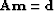whereis a vector of model parameters to be estimated,a vector of observed data and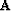a matrix or a seismic operator. The unknown model vectoris estimated such that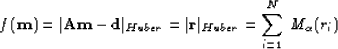(2)
is minimum. Because of the definition in equation (), althoughis linear, the minimization of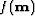in equation () is not a linear problem: a particular point in the residual can oscillate between the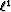andnorm for different iterations. This difficulty can be overcome by designing a nonlinear solver that will converge to a minimum of. Some specially adapted Huber minimizers have been suggested Ekblom and Madsen (1989); Li and Swetist (1998). One of the questions for this study was whether a standard non-linear method, as opposed to a special solver, would perform satisfactorily in Huber estimation. I show that it does. In addition, these specially adapted minimizers work only whenis a matrix and not an operator.

In this Chapter, a quasi-Newton method is chosen. The quasi-Newton method is an iterative process where the solution to the problem is updated as follows:(3)
where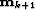is the updated solution at iteration k+1,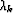the step length computed by a line search that ensures a sufficient decrease ofand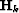an approximation of the Hessian (or second derivative.)

Quasi-Newton methods intend to approximate the Hessian without explicitely computing it. The Hessian is then re-estimated and improved at each iteration of the descent method Gill et al. (1986). Quasi-newton methods can be equivalent to conjugate-gradient on a quadratic function and they are often more robust than conjugate-gradient on general nonlinear problems. They also tend to require less iterations Gill et al. (1986). However, one major drawback of the quasi-Newton methods is that they require the storage of the approximate Hessian in memory, which can be troublesome for large scale problems.

In Appendix, a computationally effective method that does not require the storage of the Hessian is presented. This method is called limited-memory BFGS (L-BFGS) Nocedal (1980). With this technique, only a limited number of solution step and gradient step vectors are stored as the iterations go on. For each new iteration, these vectors are combined to form the approximate Hessian (see Appendixfor details). In the case where all the solution step and gradient step vectors are kept in memory at every iteration, the L-BFGS method becomes equivalent to the BFGS update Nocedal (1980). With the L-BFGS method, the storage needed is reduced compare to BFGS and makes it affordable to use for image estimation.

One computational burden is the line search algorithm that ensures sufficient decrease of the misfit function. This comes from the need to evaluate the misfit function many times before finding a good step length.The value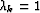is tested first Liu and Nocedal (1989), thus saving substantial computational time.

Another source of improvement is by scaling the Hessian at each iteration Liu and Nocedal (1989). This scaling greatly improves the performances of the quasi-Newton method. Liu and Nocedal (1989) show on numerical examples for large-scale problems that the L-BFGS method with the scaling of the Hessian and an appropriate line search algorithm (see Appendixfor details) is usually faster than conjugate-gradient using the Polak-Ribière formula Kelley (1999).

One problem with using quasi-Newton method, however, is that the Huber function is not twice continuously differentiable. This assumption is at the heart of the convergence properties of the L-BFGS method. Nonetheless, the L-BFGS update requires the computation of the gradient only (see Appendix). Furthermore, given that the approximate Hessian is not an exact representation of the real one, the violation to this initial condition are expected to be mild. Examples in the next section show that the method is never-the-less giving satisfying results and is robust to outliers, as expected.

In this section, I have proposed an efficient algorithm that will minimize any misfit function using the Huber norm. This algorithm is a limited-memory BFGS technique that saves computational time and memory requirement by (1) limiting the number of vectors kept in memory for the update of the Hessian, (2) testing a default value for the step length, and (3) scaling the Hessian at each iteration. In the next section, this algorithm is tested on a geophysical problem and compare the Huber norm with thenorm with and without regularization.Next: Application of the Huber Up: Robust inversion using the Previous: Introduction
Stanford Exploration Project
5/5/2005